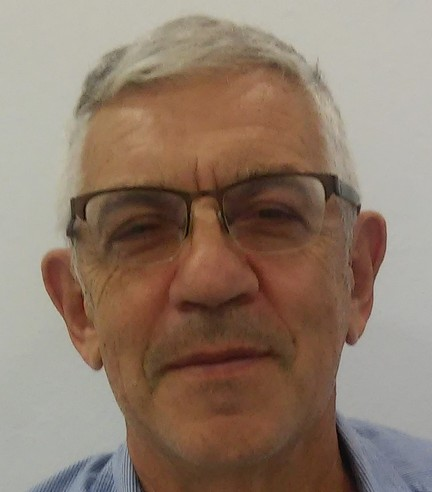# ד"ר פבל סטיאנוב

I work as a lecture and researcher in Sami Shamoon College of Engineering (since its foundation in 1995). My interests include tertiary education, pedagogical strategies for developing creative mathematical thinking, graphical approaches for teaching calculus and the application of scientific calculators and computer programs in mathematics education### פרופיל

היחידה למתמטיקה
מרצה בכיר
באר שבעב"ש

106ה איינשטיין (40)## השכלה

Ph.D.  -  1979-1982                    Russian State Pedagogical University. St Petersburg.

Name of advisor: Prof. Myshkis, A.

Name of Thesis: “The Use of  Problems with  Graphs  in Teaching  of  Calculus”.

M.Sc    - 1968 -1970                  Novosibirsk State University, Department of Mathematics.

Name of advisor: Dr. Besedin, B.

Name of Thesis: "Optimal Decision Process for One Specific Stochastic System".

B.Sc.  - 1965 -1968                 Novosibirsk State University, Department of Mathematics.

## תחומי מחקר

Different aspects of Mathematics Education, psychology of concept formation, strategies for developing of creative mathematical thinking, graphical approaches and the applications of scientific calculators and computer programs in mathematics education.

## קורסים

Calculus 1 (all levels)
Calculus 2 (all levels)
Ordinary Differential equations
Linear Algebra
Functions of Complex Variable.
The Development of Mathematical Thinking